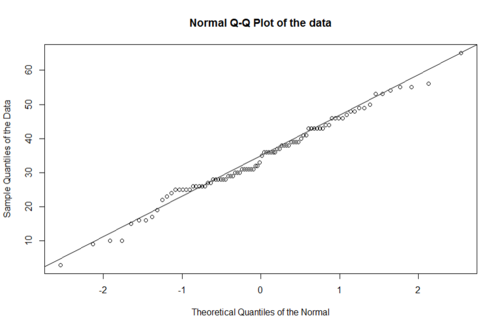# SMHS DataSimulation

## Scientific Methods for Health Sciences - Data Simulation

### Importing observed data for exploratory analytics

```# data_1 <- read.csv('00_Tiny_SOCR_HELP_Data_Simmulation.csv',as.is=T, header=T)
```
```attach(data_1)
# to ensure all variables are accessible within R, e.g., using age instead of data_1\$\\$\$age
# i2 maximum number of drinks (standard units) consumed per day (in the past 30 days range 0–184) see also i1
# treat randomization group (0=usual care, 1=HELP clinic)
# pcs SF-36 Physical Component Score (range 14-75)
# mcs SF-36 Mental Component Score(range 7-62)
# cesd Center for Epidemiologic Studies Depression scale (range 0–60)
# indtot Inventory of Drug Use Con-sequences (InDUC) total score (range 4–45)
# drugrisk Risk-Assessment Battery(RAB) drug risk score (range0–21)
# satreat any BSAS substance abuse treatment at baseline (0=no,1=yes)

==='"`UNIQ--h-2--QINU`"'Fragment of the data===

<center>
{| class="wikitable" style="text-align:center; " border="1"
|-
! ID ||i2 ||age ||treat ||homeless ||pcs ||mcs ||cesd ||indtot ||pss_fr ||drugrisk ||sexrisk ||satreat ||female ||substance ||racegrp
|-
| 1 ||0 ||25 ||0 ||0 ||49 ||7 ||46 ||37 ||0 ||1 ||6 ||0 ||0 ||cocaine ||black
|-
| 2 ||18 ||31 ||0 ||0 ||48 ||34 ||17 ||48 ||0 ||0 ||11 ||0 ||0 ||alcohol ||white
|-
| 3 ||39 ||36 ||0 ||0 ||76 ||9 ||33 ||41 ||12 ||19 ||4 ||0 ||0 ||heroin ||black
|-
| … || || || || || || || || || || || || || || ||
|-
| 100 ||81 ||22 ||0 ||0 ||37 ||17 ||19 ||30 ||3 ||0 ||10 ||0 ||0 ||alcohol ||other
|}
</center>

==='"`UNIQ--h-3--QINU`"'Testing section===

summary(data_1)

x.norm <- rnorm(n=200, m=10, sd=20)
hist(x.norm, main="N(10,20) Histogram")
hist(x.norm, main="N(10,20) Histogram")
mean(data_1\$\\$\$age)
sd(data_1\$\\$\$age)
```

## Simulate New Data to Match the Properties/Characteristics of Observed Data

• i2 [0: 184]
• age m=34,sd=12
• treat {0,1}
• homeless {0,1}
• pcs 14-75
• mcs 7-62
• cesd 0–60
• indtot 4-45
• pss_fr 0-14
• drugrisk 0-21
• sexrisk
• satreat (0=no,1=yes)
• female (0=no,1=yes)
• racegrp (black, white, other)
```# Demographics variables
Sex <- ifelse(runif(NumSubj)<.5,0,1)
Weight <- as.integer(rnorm(NumSubj, 80,10))
Age <- as.integer(rnorm(NumSubj, 62,10))
```
```# Diagnosis:
Dx <- c(rep("PD", 100), rep("HC", 100), rep("SWEDD", 82))
```
```# Genetics
chr12_rs34637584_GT <- c(ifelse(runif(100)<.3,0,1), ifelse(runif(100)<.6,0,1), ifelse(runif(82)<.4,0,1))                              # NumSubj Bernoulli trials
chr17_rs11868035_GT <- c(ifelse(runif(100)<.7,0,1), ifelse(runif(100)<.4,0,1), ifelse(runif(82)<.5,0,1))                              # NumSubj Bernoulli trials
```
```# Clinical          # rpois(NumSubj, 15) + rpois(NumSubj, 6)
UPDRS_part_I <- c( ifelse(runif(100)<.7,0,1)+ifelse(runif(100)<.7,0,1),
ifelse(runif(100)<.6,0,1)+ ifelse(runif(100)<.6,0,1),
ifelse(runif(82)<.4,0,1)+ ifelse(runif(82)<.4,0,1) )
UPDRS_part_II <- c(sample.int(20, 100, replace=T), sample.int(14, 100, replace=T),
sample.int(18, 82, replace=T) )
UPDRS_part_III <- c(sample.int(30, 100, replace=T), sample.int(20, 100, replace=T),
sample.int(25, 82, replace=T) )
```
```# Time: VisitTime – done automatically below in aggregator
```
```# Data (putting all components together)
sim_PD_Data <- cbind(
rep(Cases, each= NumTime),                          # Cases
rep(L_caudate_ComputeArea, each= NumTime), # Imaging
rep(Sex, each= NumTime),                            # Demographics
rep(Weight, each= NumTime),
rep(Age, each= NumTime),
rep(Dx, each= NumTime),                             # Dx
rep(chr12_rs34637584_GT, each= NumTime),            # Genetics
rep(chr17_rs11868035_GT, each= NumTime),
rep(UPDRS_part_I, each= NumTime),                   # Clinical
rep(UPDRS_part_II, each= NumTime),
rep(UPDRS_part_III, each= NumTime),
rep(c(0,6,12,18), NumSubj)                          # Time
)
```
```# Assign the column names
colnames(sim_PD_Data) <- c(
"Cases",
"L_caudate_ComputeArea",
"Sex", "Weight", "Age",
"Dx", "chr12_rs34637584_GT", "chr17_rs11868035_GT",
"UPDRS_part_I", "UPDRS_part_II", "UPDRS_part_III",
"Time"
)
```
```# some QC
summary(sim_PD_Data)
dim(sim_PD_Data)
```

.........